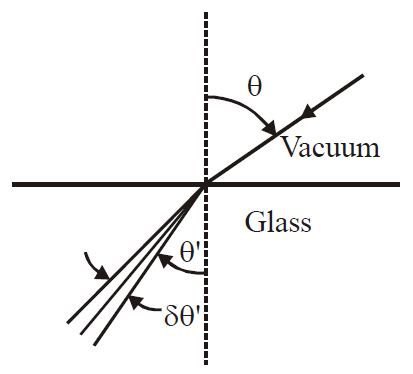# Angular width of Refracted Beam!A beam of light has a small wavelength spread $\delta \lambda$ about a central wavelength $\lambda$. The beam travels in vacuum until it enters a glass plate at an angle $\theta$ relative to the normal to the plate, as shown in the figure. The index of refraction of the glass is given by $n(\lambda)$. The angular spread $\delta \theta '$ of the refracted beam is given by?

×